# Tower Of Hanoi using Recursion in C

## Tower Of Hanoi using Recursion in C with Examples

In this article, I am going to discuss the Tower of Hanoi using Recursion in C Language with Examples. Please read our previous article, where we discussed Fibonacci Series using Recursion in C Language with Examples.  Let’s first understand what is the Tower of Hanoi problem.

##### What is the Tower of Hanoi Problem?

In this problem, we have given 3 towers. In one of the towers, there are a number of discs i.e., 3. Below is an example Image.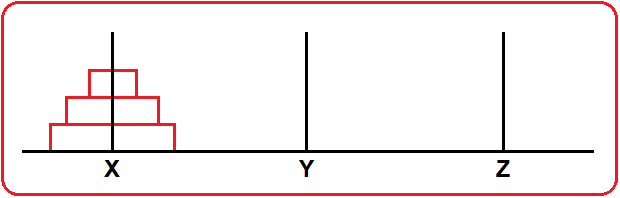In our example, we have 3 towers – X, Y, Z, and 3 discs are present in X tower. The bigger disc is kept on the bottom of the tower and the smallest disc is kept on top of the tower. Now, the problem is we have to transfer all these discs from tower X to tower Z with the following conditions –

1. We can move one disk at a time.
2. The larger disk should not be kept over the smaller disk.

We can’t keep a larger disc on a smaller disc. For moving the Discs from tower X to tower Z we required one more extra tower Y as an auxiliary tower. The following Images will help you to clearly understand this problem.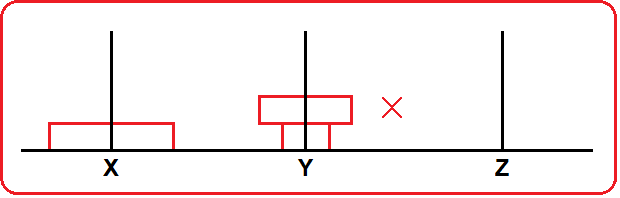Step1. We have our 3 discs in tower X.Step2. We have transferred our smaller disc from x to Z tower.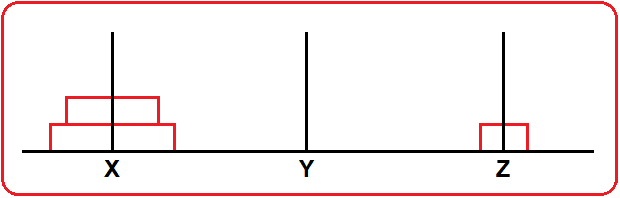Step3. Now, we have transferred our second smaller disc from X to Y.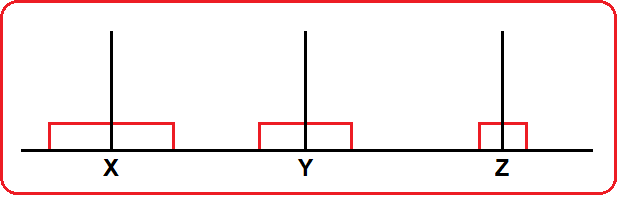Step4. Now, we have transferred our smaller disc from Z to Y. (smaller disc on top of the second larger disc)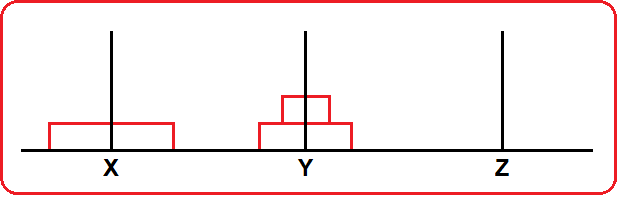Step5. Now, we have transferred our larger disc from X to Z.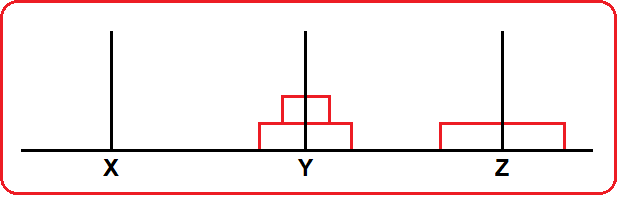Step6. Now, we have transferred our smaller disc from Y to X.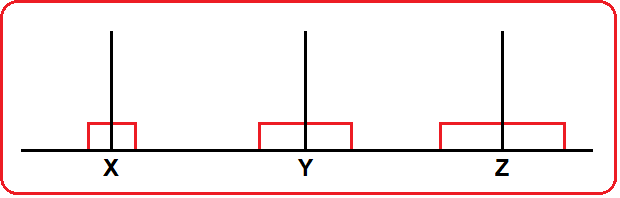Step7. Now, we have transferred our second larger disc from Y to Z.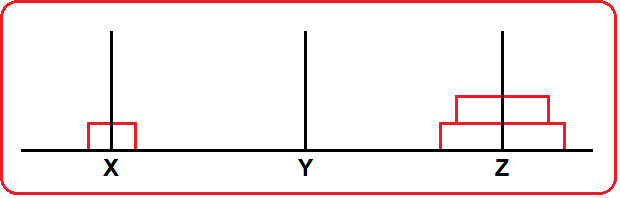Step 8. Finally, we have transferred our smaller disc from X to Z.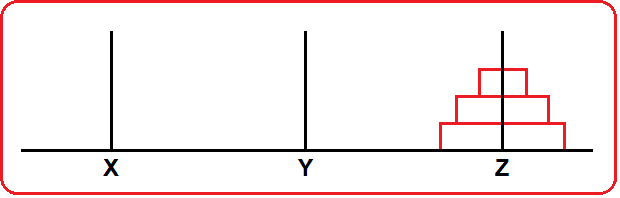Now, we want our recursive function to work in the same manner, transfer discs by following the above conditions. So, let’s have a look at our recursive function which will do the same.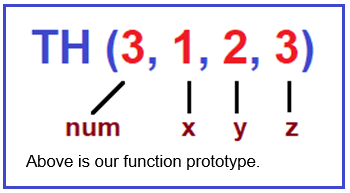##### Tower of Hanoi Program using Recursion in C Language:

Let us first see the code and then we will understand the code using the tracing tree.

```#include <stdio.h>
void TH(int num, int x, int y, int z)
{
if(num > 0)
{
TH(num - 1, x, z, y);
printf("From %d to %d \n", x, z);
TH(num - 1, y, x, z);
}
}

int main()
{
int numOfDisc = 3;
int tower1 = 1, tower2 = 2, tower3 = 3;
TH(numOfDisc, tower1, tower2, tower3);
}
```
###### Output: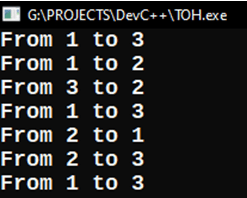Let’s understand each and every step in Tracing Tree.

#### Tracing Tree of Tower of Hanoi Program using Recursion:

##### Step1: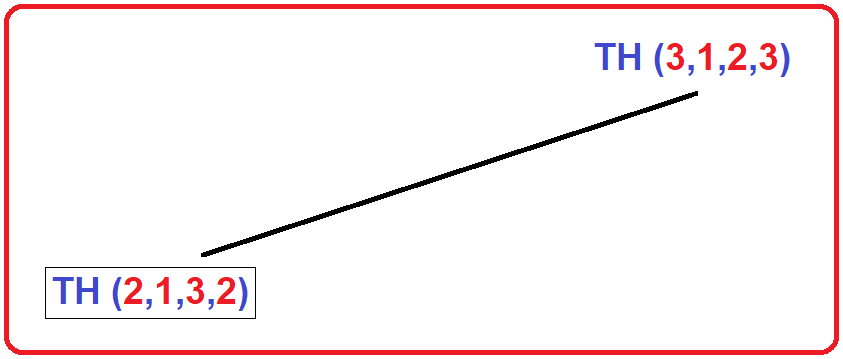We call our function with the following parameters –

num = 3, X = 1, Y = 2, Z = 3

first it will check for if (3 > 0), yes, then here are 3 things –

1. recursive call – TH (num – 1, x, z, y) (swapping of z and y)
2. print statement – printf (“From %c to %c \n”, x, z);
3. recursive call – TH (num – 1, y, x, z) (x = y, y = x, z = z)

First it has to complete its 1st recursive call then print a statement then again execute its 2nd recursive call. In above Image, it will execute TH (2, 1, 3, 2) with modified parameter as num = 2, X = 1, Y = 3, Z = 2

##### Step2: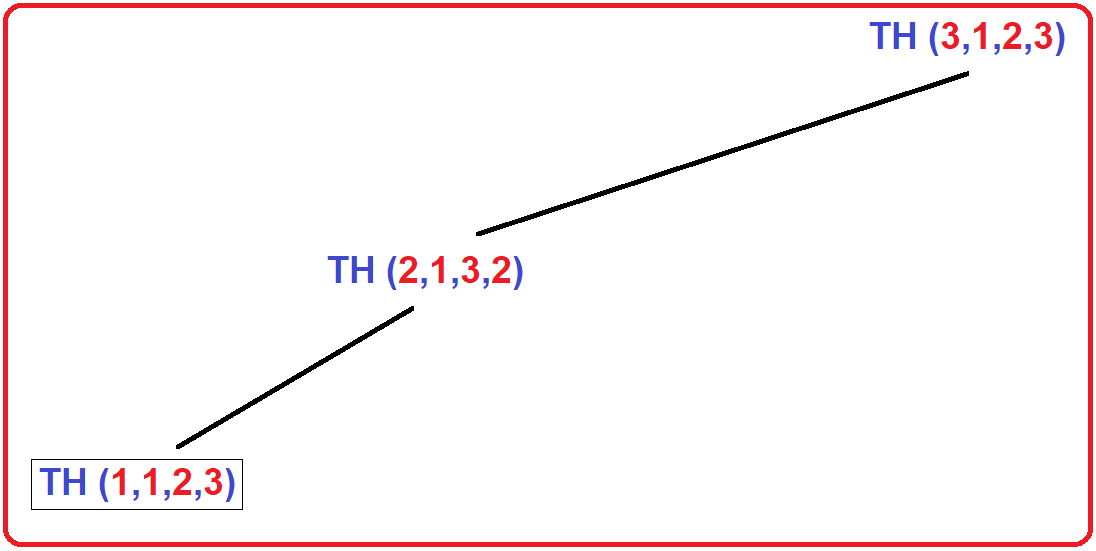Here TH (2,1,3,2) check for if (2 > 0), yes, then again here are three things- call, print and again call. It will execute its first call TH (1,1,2,3) with the modified parameter as num = 1, X = 1, Y = 2, Z = 3

##### Step3: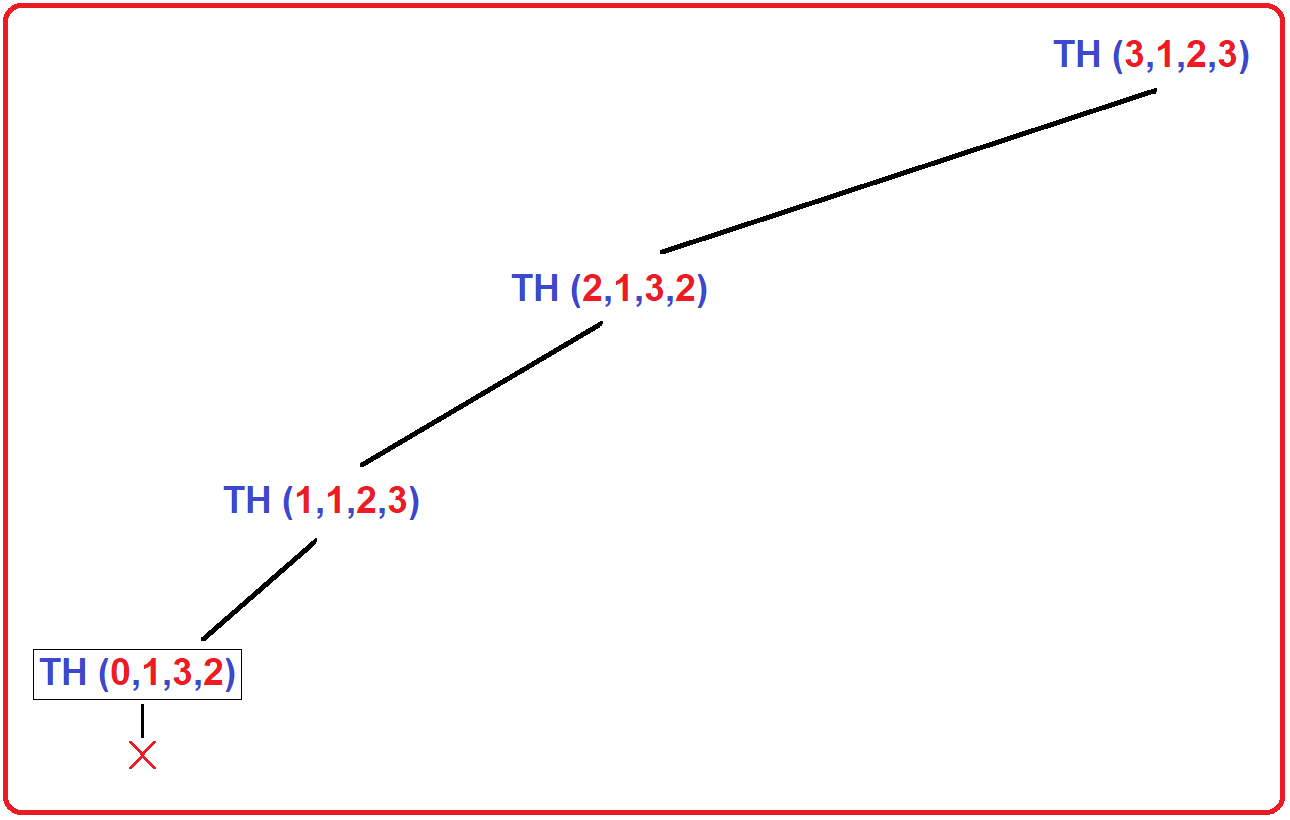Here TH (1,1,2,3) check for if (1 > 0), yes, then again here are three things- call, print and again call. It will execute its first call TH (0,1,3,2) with the modified parameter as num = 0, X = 1, Y = 3, Z = 2

##### Step4: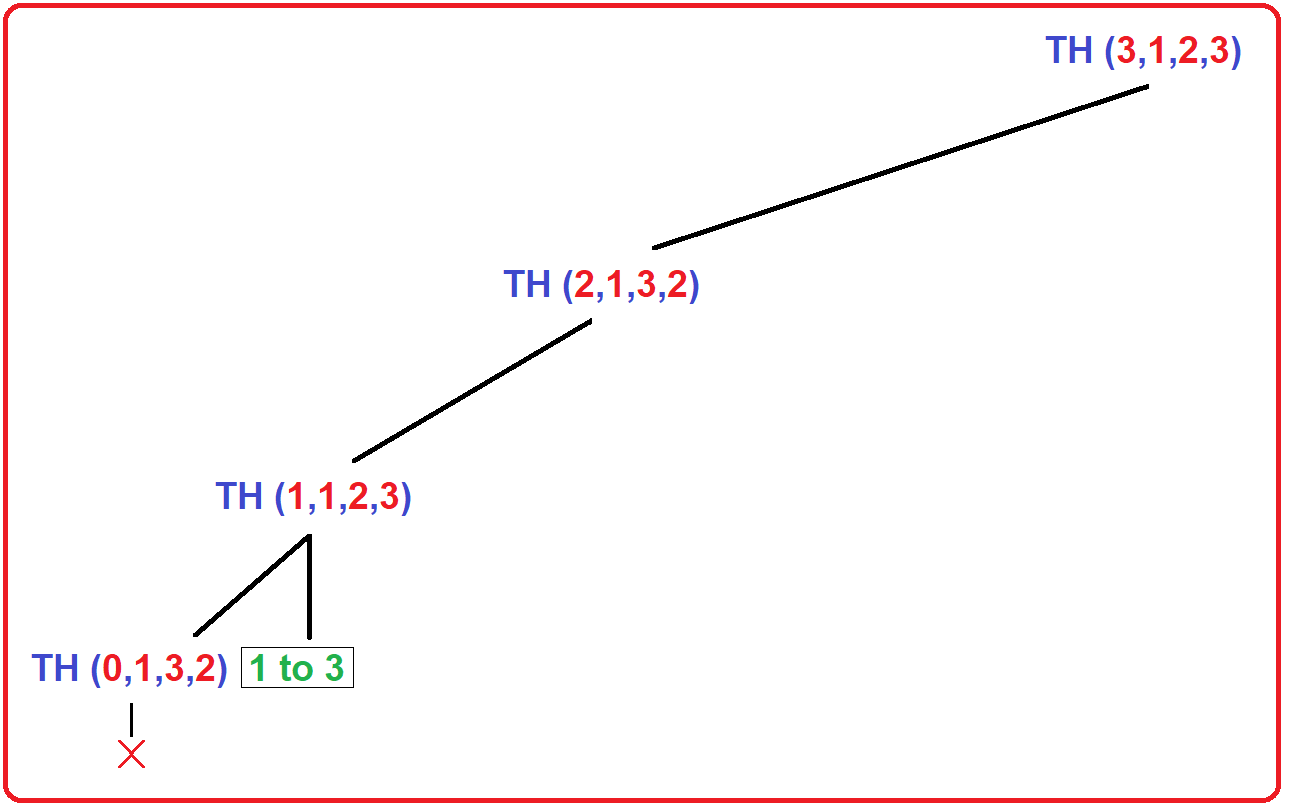TH (0,1,3,2) will check for if (0 > 0), No, this call will terminate here. Then control goes back to the previous call TH (1,1,2,3), here it has finished its first call, now it will print “1 to 3” on the screen.

##### Step5: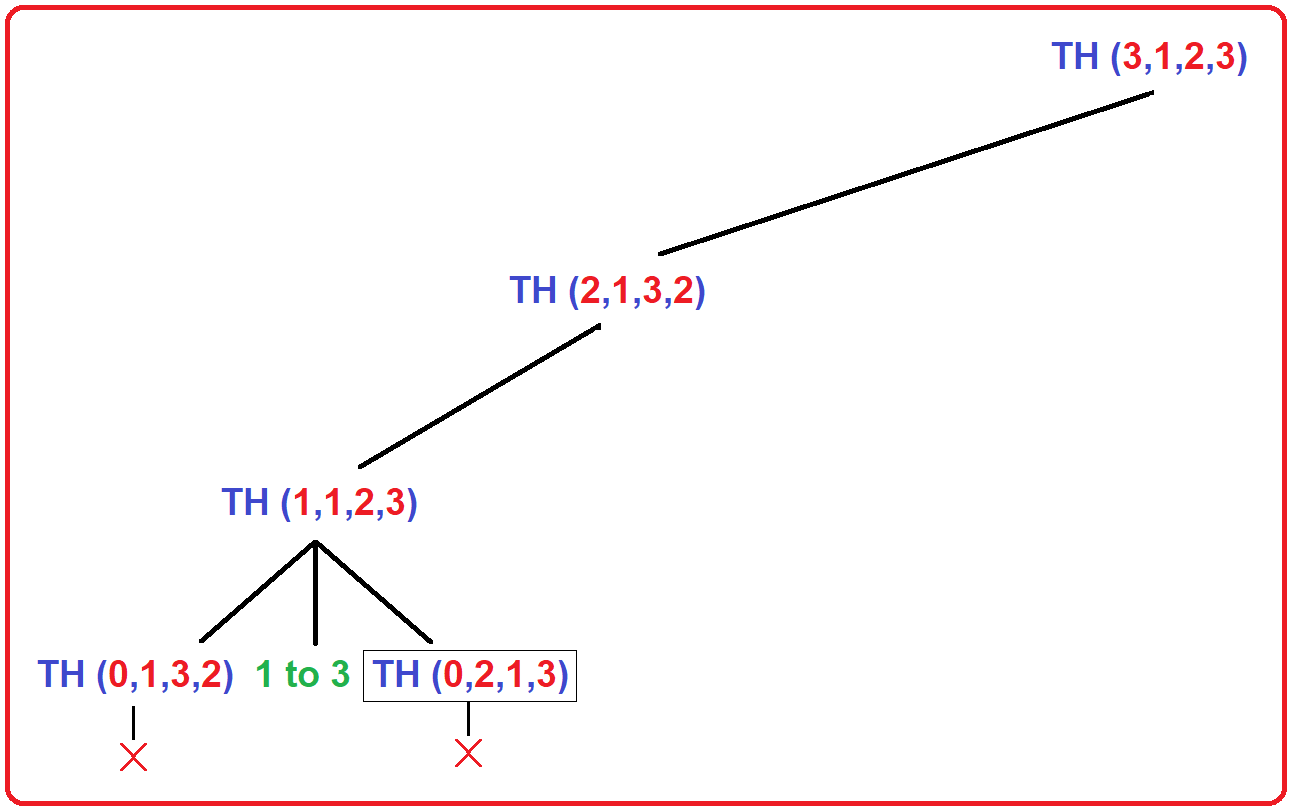After print the statement on screen, TH (1,1,2,3) will execute its 2nd recursive call which is TH (0,2,1,3) with the modified parameters as: num = 0, X = 2, Y = 1, Z = 3.

Inside this call, it will check for if (0 > 0), No, it will terminate here and control goes back to previous call TH (1,1,2,3). As this call is completed its all 3 steps, control return to the previous call which is TH (2,1,3,2).

##### Step6: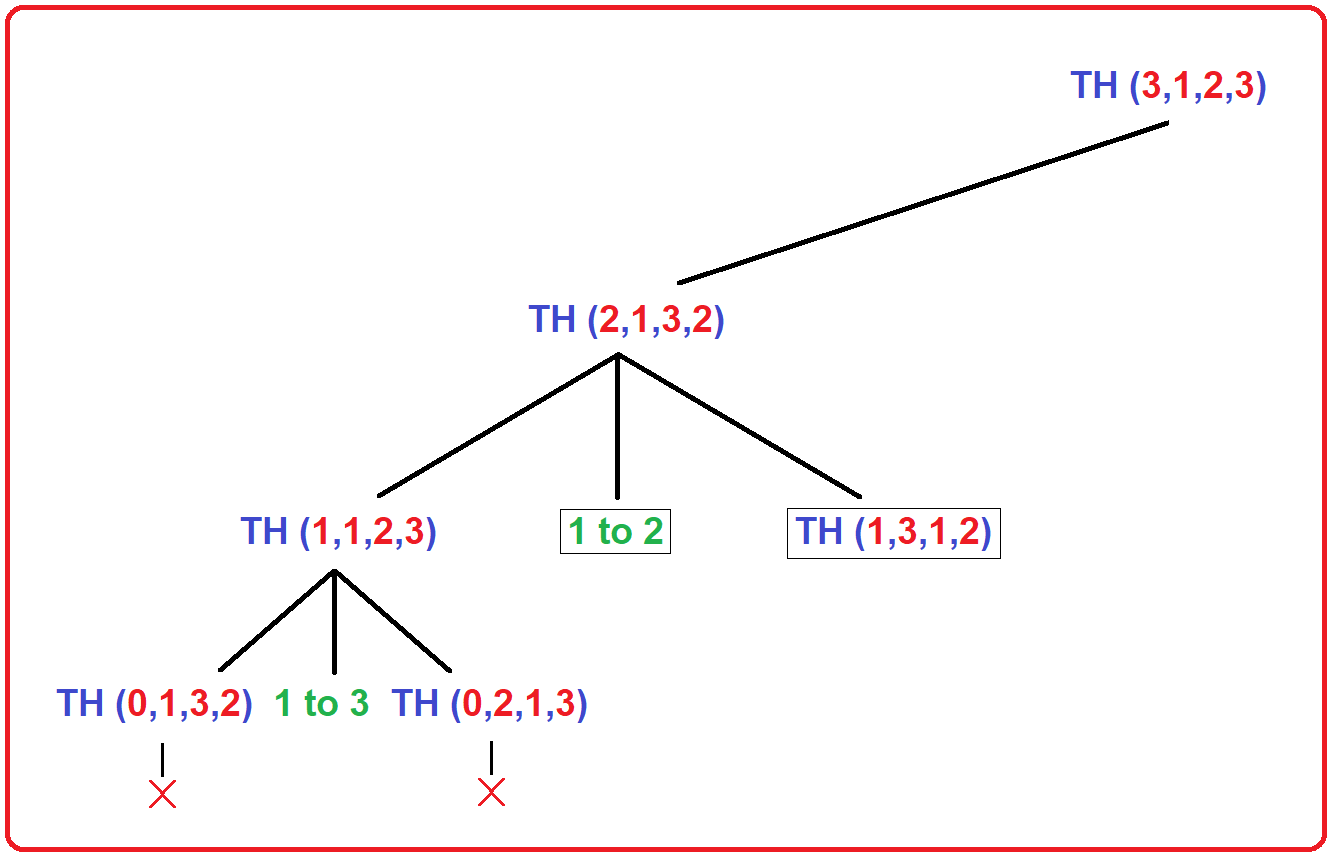As shown in above Image, TH (2,1,3,2) has completed its first call, now it will print “1 to 2” on the screen. After printing, it will execute its 2nd recursive call TH (1,3,1,2) with the following modified parameters: num = 1, X = 3, Y = 1, Z = 2.

##### Step7: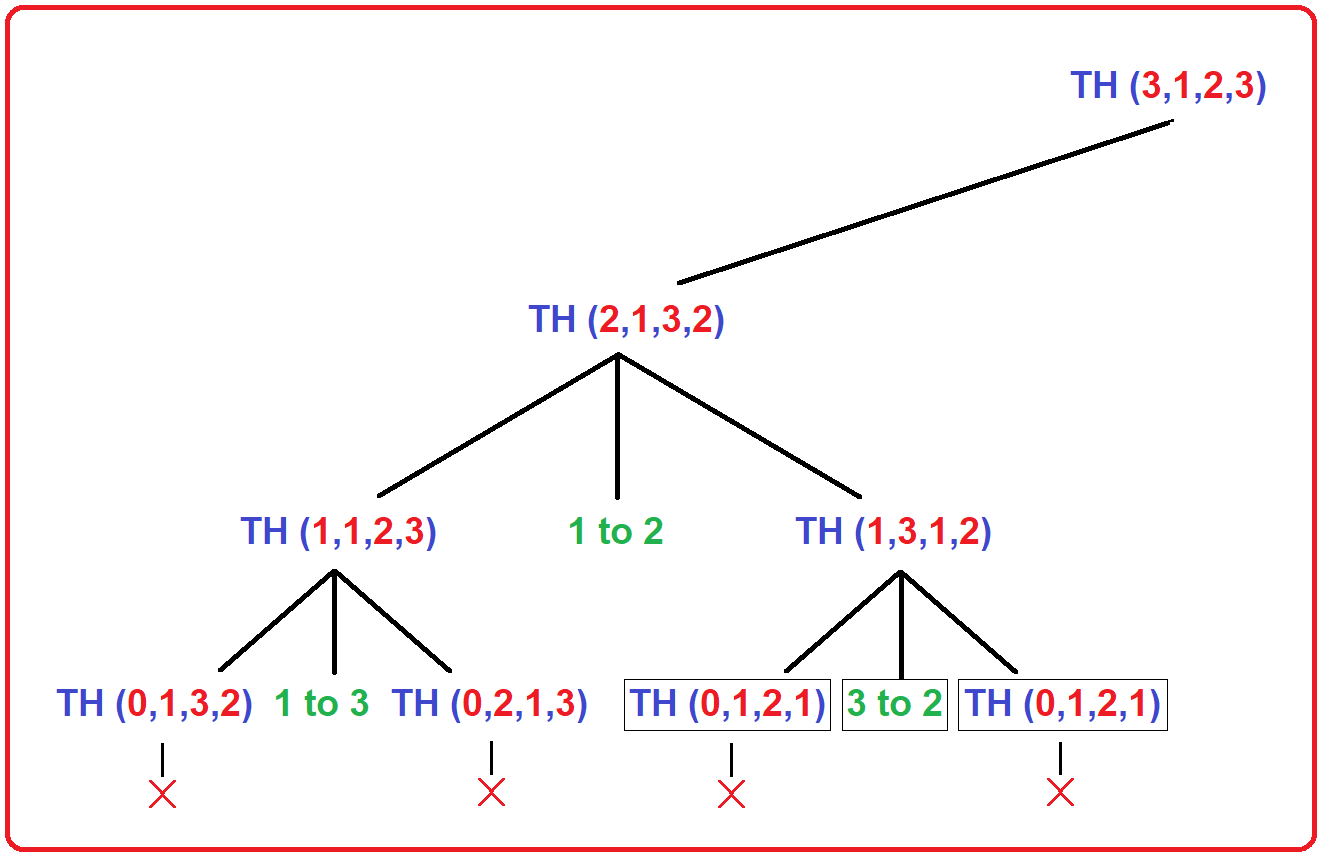Here, TH (1,3,1,2) will execute all 3 steps as you can see in the above image. The first call will terminate because it will fail for if condition. Then it will print “3 to 2” on the screen and after that, it will call its 2nd recursive call which will also terminate hare.

Now, here, all steps of call TH (1,3,1,2) have finished. Here, control goes back to the previous call TH (2,1,3,2) which also executed all 3 steps, again control goes back to the previous call which is TH (3,1,2,3).

##### Step8: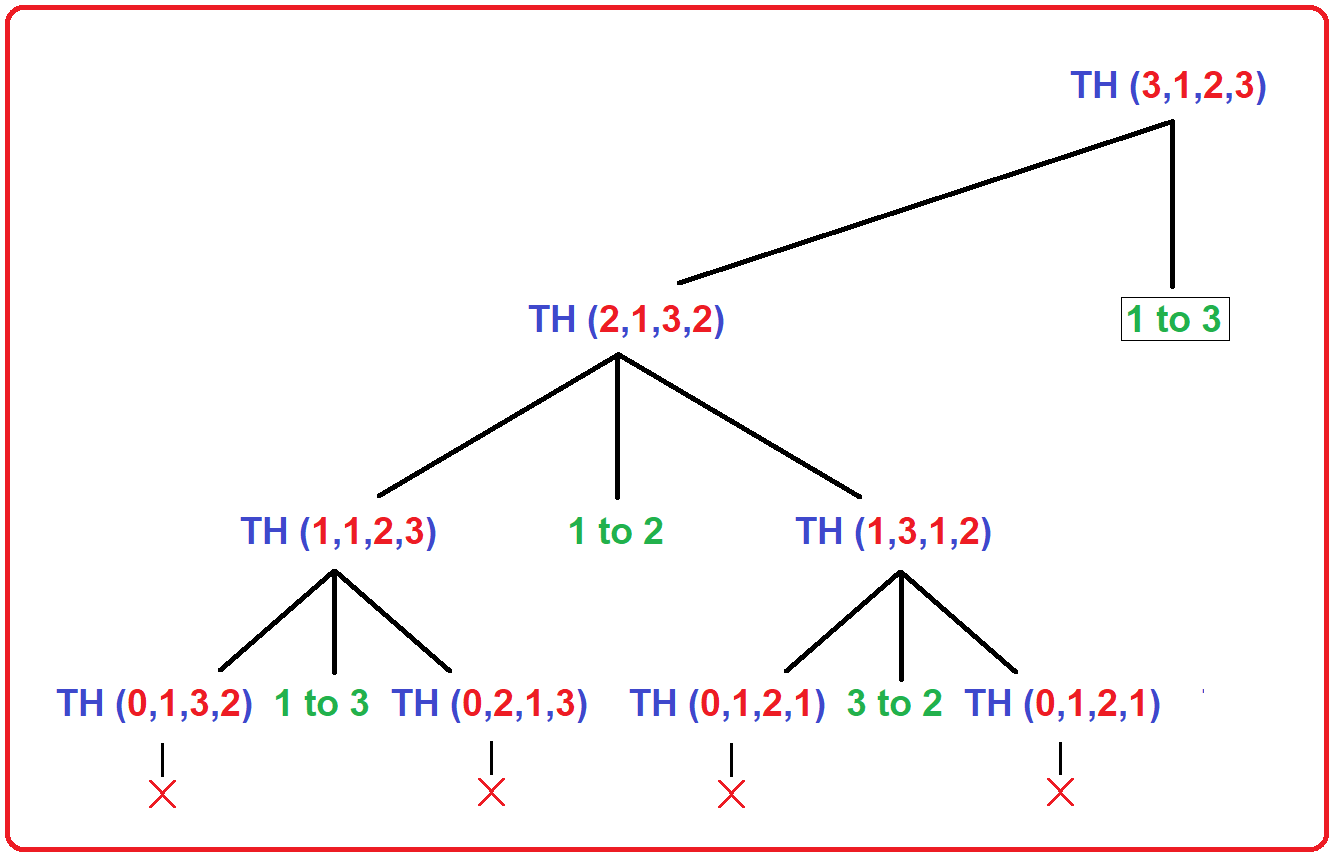As seen in Image, TH (3,1,2,3) completed its first call, now it will print “1 to 3” on the screen.

##### Step9: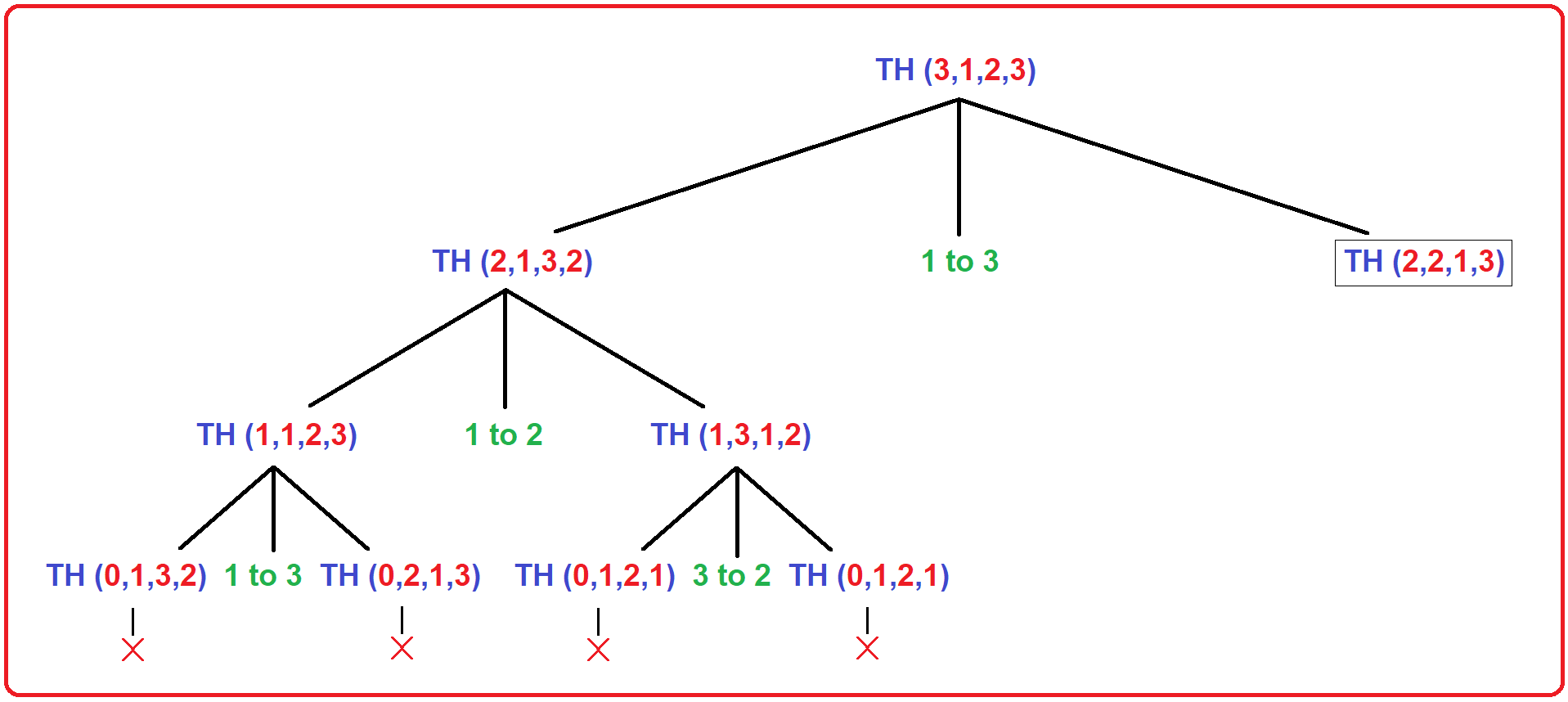After print the statement, TH (3,1,2,3) will execute its 2nd recursive call which is TH (2,2,1,3).

##### Step10: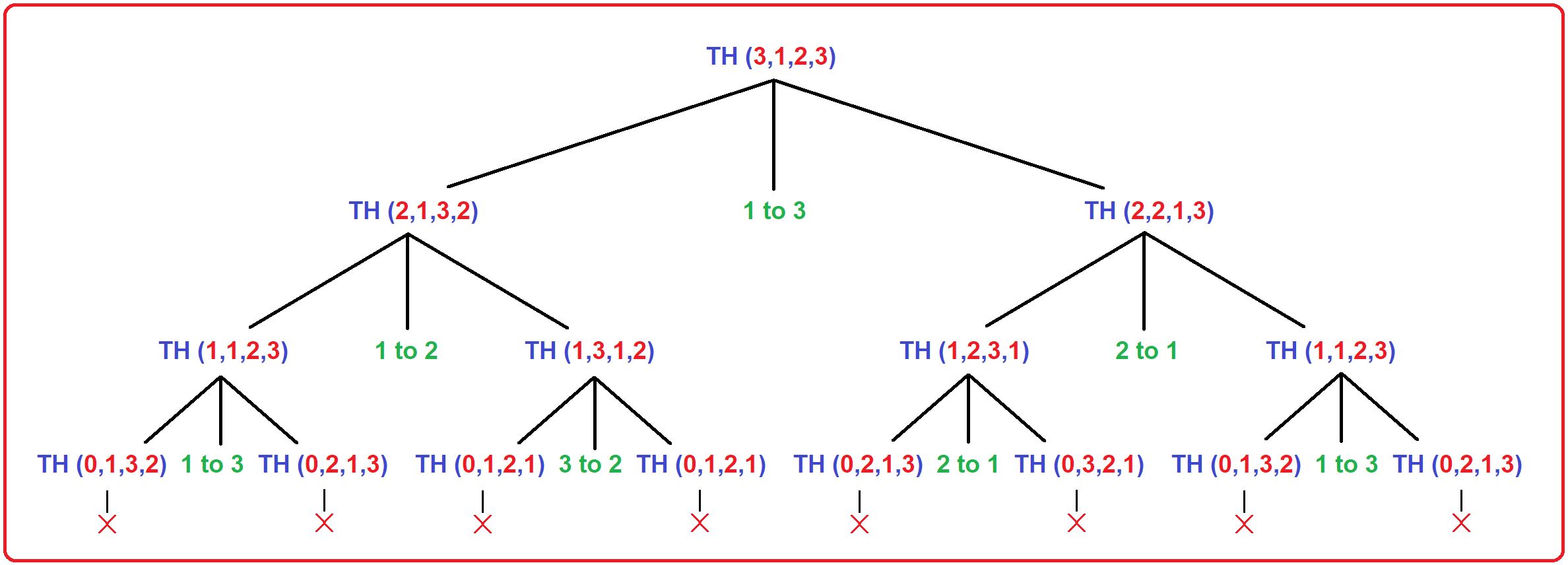As seen in Image, TH (2,2,1,3) will complete all 3 steps in the same manner as we discussed in previous calls, and at last, it will terminate and the whole call will finish.

Here, in this article, I try to explain the Tower of Hanoi using Recursion in C Language with Example and I hope you enjoy this Tower of Hanoi using Recursion in C Language with Example article.